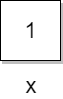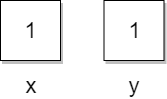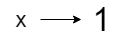## Python Programming Tutorial Index

Before understanding the concept of variables in Python, you must know how variables work in other programming languages. In C, Java, and many other programming languages, the concept of a variable is linked to memory space, and a variable is perceived as a storage box that can store some value.

Here's an example of how the concept of variables works in other programming languages:

Example:

``x = 1;``

In the above example, memory is allocated with the name "`x`", and the value "`1`" is stored there. Here, we can see the memory as a container that holds the value, as shown in the picture below.This way, for each variable, there will be a new memory space created with the variable's name, and if we change the variable's value, then memory will be updated with the new value.

Example:

``int x,y = 1;``This lets us understand how the variable works in other programming languages. But in Python, the case is different, and here a variable is seen as a tag or name tied to some value. Here is an example of how to declare a variable in Python:

Example:

``x = 1``

In the above Python example, a value "`1`" is created in the memory, and then the tag name "`x`" has been created, which is tied to the value.If we change the variable value to a new value, a new value is created in memory, and the tag is shifted to a new one. The old value becomes un-referenced in this case, and the garbage collector removes it.

Assigning a variable to another variable creates a new tag connected to the same value.Example:

``int y = x;``

In the above example, we store the value of "`x`" in "`y`". A new tag, "`y`" will be generated, referring to the value "`1`".

In this way, we can understand how variables in Python differ from other programming languages.